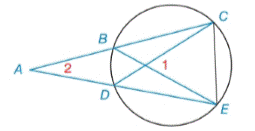Chapter 6.2, Problem 16EElementary Geometry For College St...

7th Edition
Alexander + 2 others
ISBN: 9781337614085

Solutions

Chapter
SectionElementary Geometry For College St...

7th Edition
Alexander + 2 others
ISBN: 9781337614085
Textbook Problem

Given: m ∠ 1 = 71 ° m ∠ 2 = 33 ° Find: m C E ⌢ and m B D ⌢Exercises 16, 17

To determine

To find:

mCE and mBD when m1=71°,m2=33°

Explanation

mCE=xmBD=y

Theorem:

The measure of an angle formed by two chords that intersect within a circle is one-half the sum of the measures of the arcs intercepted by the angle and its vertical angle.

mCE and mBD when m1=71°,m2=33°

By Theorem

m1=12(mCE+mBD)71°=12(x+y)x+y=2(71°)x+y=142°

Theorem:

The measure of an angle formed when two secants intersect at a point outside the circle is one-half the difference of the measures of the two intercepted arc

Still sussing out bartleby?

Check out a sample textbook solution.

See a sample solution

The Solution to Your Study Problems

Bartleby provides explanations to thousands of textbook problems written by our experts, many with advanced degrees!

Get Started

59.45 is 41% of what number?

Elementary Technical Mathematics

Write the sum in expanded form. 8. j=nn+3j2

Single Variable Calculus: Early Transcendentals

True or False: All the hypotheses for the Mean Value Theorem hold for f(x) = 1 − |x| on [−1, 2].

Study Guide for Stewart's Single Variable Calculus: Early Transcendentals, 8th